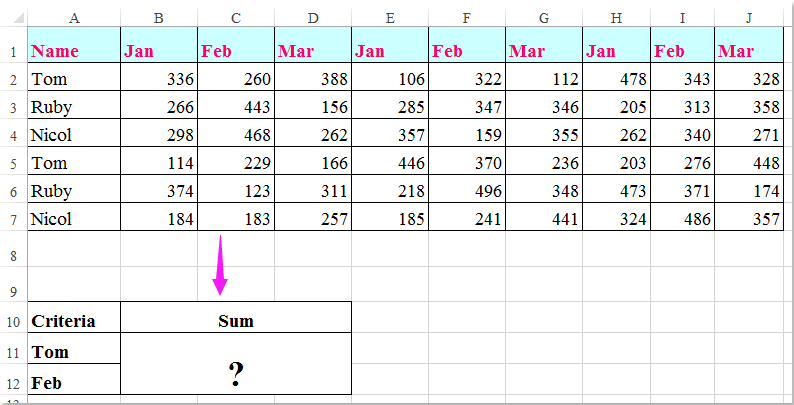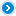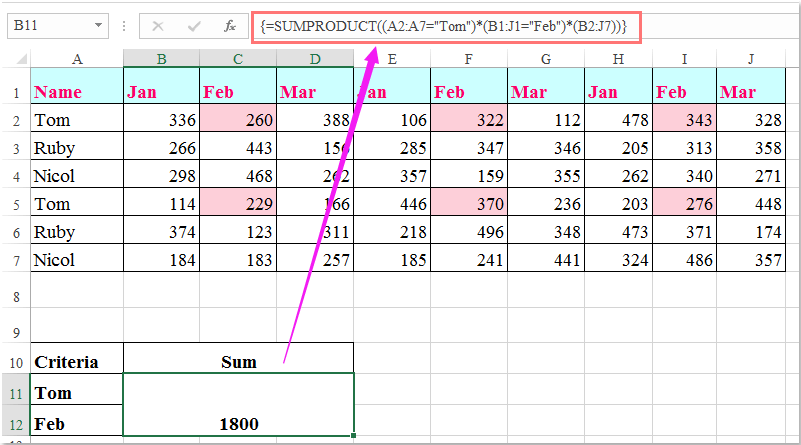Note: The other languages of the website are Google-translated. Back to English

## 如何在Excel中基于列和行条件求和？####基于列和行条件以及公式的求和单元格

=SUMPRODUCT((A2:A7="Tom")*(B1:J1="Feb")*(B2:J7))

=SUM(IF(B1:J1="Feb",IF(A2:A7="Tom",B2:J7)))### 最佳办公效率工具

#### Kutools for Excel解决了您的大多数问题，并使您的生产率提高了80％

• 重用: 快速插入 复杂的公式，图表 以及您以前使用过的任何东西； 加密单元 带密码 创建邮件列表 并发送电子邮件...
• 超级公式栏 （轻松编辑多行文本和公式）； 阅读版式 （轻松读取和编辑大量单元格）； 粘贴到过滤范围...
• 合并单元格/行/列 不会丢失数据； 拆分单元格内容； 合并重复的行/列...防止细胞重复； 比较范围...
• 选择重复或唯一 行; 选择空白行 （所有单元格都是空的）； 超级查找和模糊查找 在许多工作簿中； 随机选择...
• 确切的副本 多个单元格，无需更改公式参考； 自动创建参考 到多张纸； 插入项目符号，复选框等...
• 提取文字，添加文本，按位置删除， 删除空间; 创建和打印分页小计； 在单元格内容和注释之间转换...
• 超级滤镜 （将过滤方案保存并应用于其他工作表）； 高级排序 按月/周/日，频率及更多； 特殊过滤器 用粗体，斜体...
• 结合工作簿和工作表; 根据关键列合并表； 将数据分割成多个工作表; 批量转换xls，xlsx和PDF...
• 超过300种强大功能. 支持 Office / Excel 2007-2021 和 365。支持所有语言。 在您的企业或组织中轻松部署。 完整功能 30 天免费试用。 60 天退款保证。#### Office选项卡为Office带来了选项卡式界面，使您的工作更加轻松

• 在Word，Excel，PowerPoint中启用选项卡式编辑和阅读，发布者，Access，Visio和Project。
• 在同一窗口的新选项卡中而不是在新窗口中打开并创建多个文档。
• 每天将您的工作效率提高50％，并减少数百次鼠标单击！=SUMPRODUCT((A2:A7="Tom")*(B1:J1="Feb")*(B2:J7))

=SUMPRODUCT((A2:A7="Tom")*((B1:J1="Feb")+(B1:J1="Mar"))*(B2:J7)).

=ArrayFormula(SUM(IF(regexmatch(O5:W5,"^Fe."),IF(regexmatch(N6:N11,"^To."),O6:W11))))

ArrayFormula(SUM(IF(正则表达式(D12,""),K12:AH12)))

=SUMPRODUCT((A2:A7=A2)*(B1:J1=C1)*(B2:J7))
=SUM(IF(B1:J1=C1,IF(A2:A7=A2,B2:J7)))

=SUMPRODUCT((Column = Criteria)* ( Row = Criteria) * (第二列 = Criteria) * (Matrix) * (Matrix >0))

=SUM(IF('[Primary Controller Active Project Database (KC notes)1.xlsx]Active Project Tracking'!\$B2:\$B66=X2,IF('[Primary Controller Active Project Database (KC notes)1.xlsx]活动项目跟踪'!\$A1:\$AV1=AH10,'[主控制器活动项目数据库(KC注释)1.xlsx]活动项目跟踪'!\$H\$2:\$AV\$66)))

0   产品特性

## 关注我们

Microsoft和Office徽标是Microsoft Corporation在美国和/或其他国家的商标或注册商标。